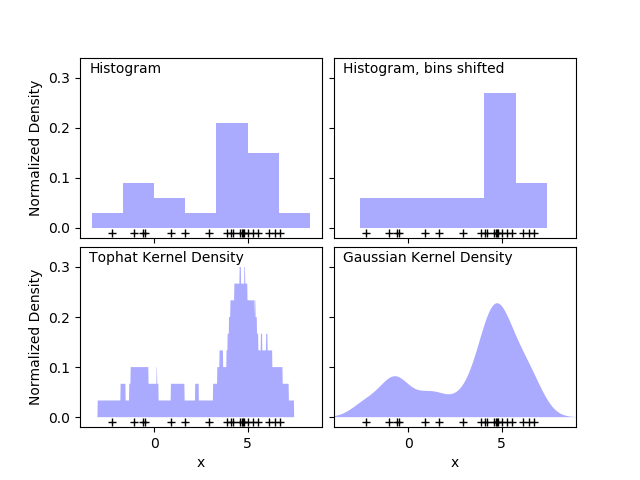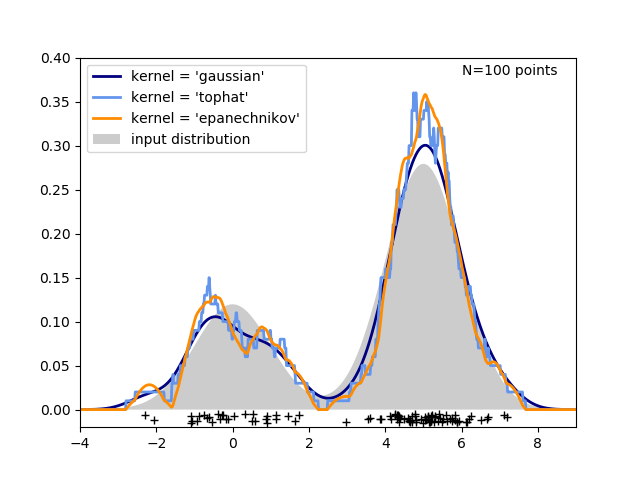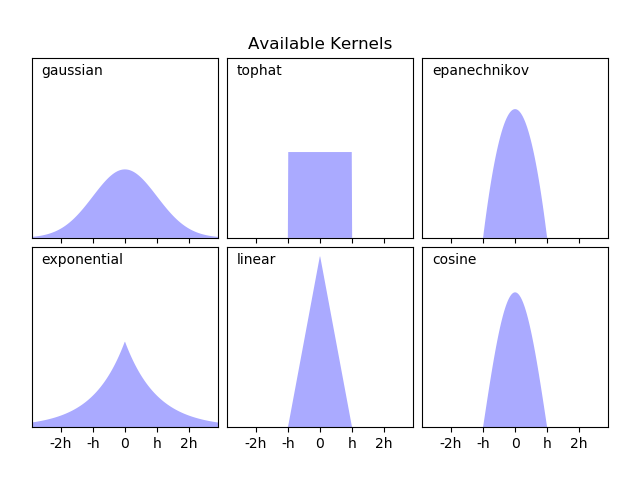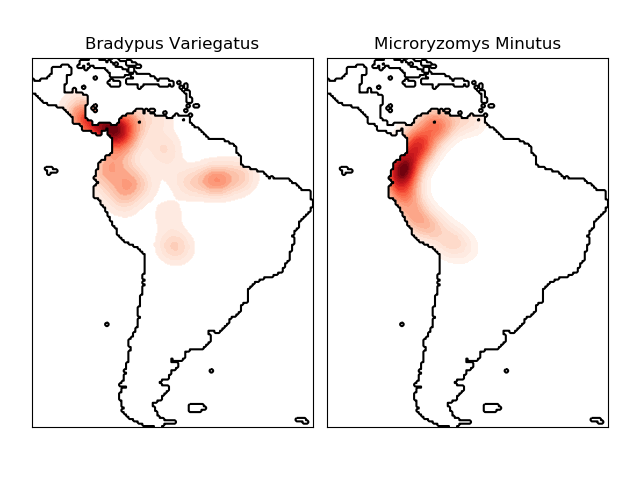# 2.8. 密度估计¶

## 2.8.1. 密度估计: 直方图然而，直方图的一个主要问题是分组的选择可能会对结果的可视化产生不成比例的影响。相同数据下,右上角的图是左上角的图向右移动后产生的。两种可视化的结果看起来完全不同，可能会导致对数据不同的解释。

## 2.8.2. 核密度估计

scikit-learn中的核密度估计是在sklearn.neighbors.KernelDensity估计器中实现的，该估计器使用Ball Tree或KD Tree进行高效查询（有关这些内容的讨论，请参阅最近邻）。尽管为简化起见，上面的示例使用一维数据集，但是可以在任意多个维度上执行核密度估计，不过在实践中，维度灾难会导致其性能在高维度上下降。很明显，核形状如何影响结果分布的平滑度。scikit-learn核密度估计器可以按以下方式使用：

>>> from sklearn.neighbors import KernelDensity>>> import numpy as np>>> X = np.array([[-1, -1], [-2, -1], [-3, -2], [1, 1], [2, 1], [3, 2]])>>> kde = KernelDensity(kernel='gaussian', bandwidth=0.2).fit(X)>>> kde.score_samples(X)array([-0.41075698, -0.41075698, -0.41076071, -0.41075698, -0.41075698,       -0.41076071])

sklearn.neighbors.KernelDensity 实现几种常见形状的的核，如下图所示：这些核的形状如下所示:

• Gaussian kernel ( kernel = 'gaussian' )

• Tophat kernel ( kernel = 'tophat' ) if $x • Epanechnikov kernel ( kernel = 'epanechnikov' ) • Exponential kernel ( kernel = 'exponential' ) • Linear kernel ( kernel = ' linear' ) if$x

• cosine kernel ( kernel = 'cosine' ) 如果 \$x核密度估计的另一个有用的应用是从数据集中学习出一个非参数生成模型，以便有效地从该生成模型中绘制新的样本。这是一个示例，该示例使用高斯核对数据的 PCA 投影进行学习，创建一组新的手写数字的示例，：

“新”数据由输入数据线性组合而成，其权重根据 KDE 模型按概率给出。